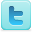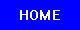Mathematics
Science Fair Projects
Ideas and Sample Projects by Grade Level

Popular Project Ideas
P=Project   E=Experiment
Is Mathematics Discovered or Invented? [E]
Exploration and Analysis of Highly Divisible Numbers (antiprimes) [P]
Tetrahedral Shoelace Algorithm: Calculating Volume of Irregular Solids [P]
Use algebra and geometry to calculate the relative probability of making a successful bank shot from different positions on the court, keeping the distance to the hoop constant. Then test if you can reproduce your predictions with a scale model. [E]
A statistical estimation of the No. of headwords in a dictionary and the size of your vocabulary. [E]
Determine if flipping a coin is truly random. [E]
Does The Size Of A Circle Affect The Value Of Pi? [E]
Test the relationship between the three different dimensions (length, width and height) of a three-dimensional object with a constant volume. [E]
Measure the dimensions of different juice box products to find out which manufacturer has the largest volume of juice and uses the least amount of packaging material. [E]
Find out if playing video games can improve or hurt your math skills. [P]
Does music affect students' math performance? [P] [P]
Mathematics: Is There a Gender Difference? [E]
Measure the distance balls travel when launched from a catapult, and use the results to construct a histogram and assess the catapult's reliability. [E]
How Fractals Can Explain What's Wrong with Wall Street [E]
What Makes a Team's Winning Percentage Deviate from the Pythagorean Relationship? [E]
Devising an Algorithm for Solving Rubik's Cube [E]
Determine the best algorithm for strategy games. [E]
Discover a method to multiply two numbers represented by the same quadratic form. [E]
Research the Leibniz and Newton calculus controversy [E]

 Mathematics Topics Related Subjects Statistics & Probability Geometry & Trigonometry Applied Mathematics Games & Game Theory Number Theory Mathematics Learning & Cognition Algebra & Calculus Computer Science Intelligence

 Science Fair Project Guide Home Science Fair Project Types The Scientific Method - How to Experiment The Display Board Topics, Ideas, Sample Projects